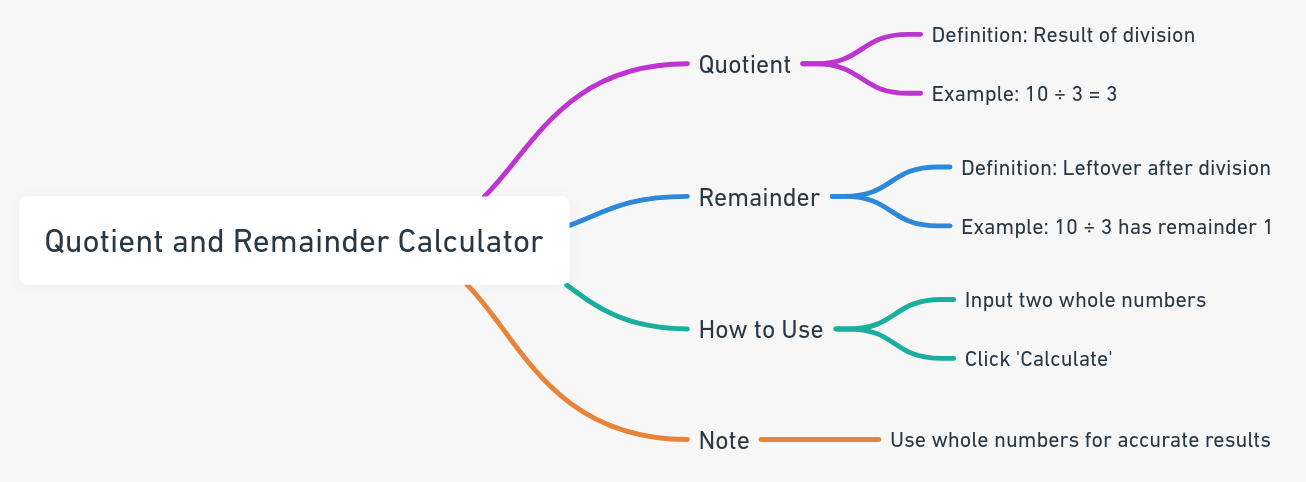Even The mini Tools Can Empower People to Do Great Things.

# Quotient and Remainder Calculator - Easily Find Quotient and Remainder

Quotient and Remainder Calculator
 Dividend: Divisor:

Embed Quotient and Remainder Calculator Widget

## About Quotient and Remainder CalculatorDividing numbers and not sure about the quotient or remainder? This tool is used to find the quotient and remainder of a division of two whole numbers Dividend and Divisor given by Dividend/Divisor = Quotient + Remainder/Divisor.

## FAQ

In arithmetic, a quotient is the quantity produced by the division of two numbers. For example, (10/3) = 3.
A remainder is the integer left over after dividing one integer by another. For example, the remainder of 10/3 is 1.
Input Dividend and Divisor and click Calculate Quotient and Remainder button. Please note you must input whole numbers on the form.
Whole numbers are integers greater or equal to 0. For example:0, 7, 81, 3897 are all whole numbers, but 1/5, 6.78, 100.00001 are not whole numbers.

## Reference:

Reference this content, page, or tool as:

"Quotient and Remainder Calculator" at https://miniwebtool.com/quotient-and-remainder-calculator/ from miniwebtool, https://miniwebtool.com/

## Related Miniwebtools:

Modulo (Mod) Calculator